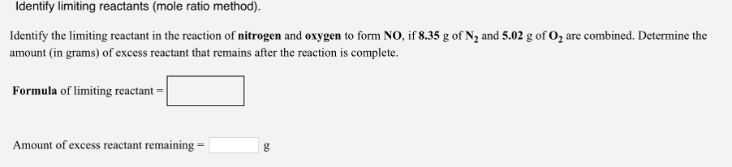# Problem: Identify limiting reactants (mole ratio method). Identify the limiting reactant in the reaction of nitrogen and oxygen to form NO, if 8.35 g of N2 and 5.02 g of O2 are combined. Determine the amount (in grams) of excess reactant that remains after the reaction is complete. Formula of limiting reactant = Amount of excess reactant remaining =

###### FREE Expert Solution
100% (300 ratings)###### Problem Details

Identify limiting reactants (mole ratio method).

Identify the limiting reactant in the reaction of nitrogen and oxygen to form NO, if 8.35 g of N2 and 5.02 g of O2 are combined. Determine the amount (in grams) of excess reactant that remains after the reaction is complete.

Formula of limiting reactant =

Amount of excess reactant remaining =